Next: 6.2 Approximation Up: 6. Limits of Sequences Previous: 6. Limits of Sequences   Index

# 6.1 Absolute Value

6.1   Definition (Absolute values.) Recall that if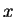is a real number, then the absolute value of, denoted by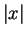, is defined by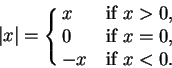We will assume the following properties of absolute value, that follow easily from the definition:

For all real numbers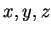with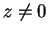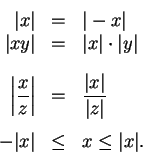For all real numbers, and all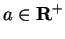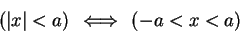and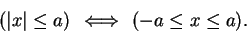(6.2)

We also have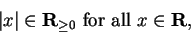and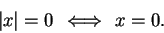6.3   Theorem. Let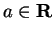and let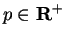. Then for all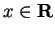we have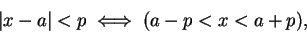and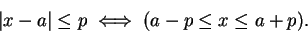Equivalently, we can say that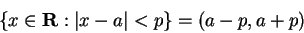and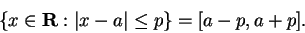Proof:    I will prove only the first statement. I have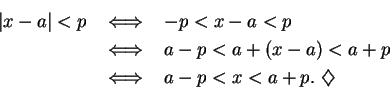6.4   Definition (Distance.) The distance between two real numbersand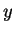is defined by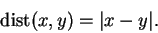Theorem 6.3 says that the set of numbers whose distance from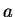is smaller than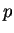is the interval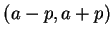. Geometrically this is clear from the picture.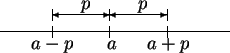I remember the theorem by keeping the picture in mind.

6.5   Theorem (Triangle inequality.) For all real numbersand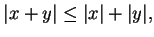(6.6)

Proof      For allandin R we have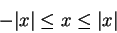and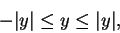so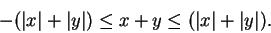Hence (Cf. (6.2))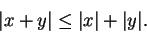6.7   Exercise. Can you prove that for all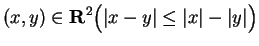?

Can you prove that for all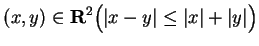?

Remark: Let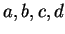be real numbers with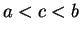and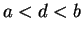.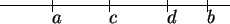Then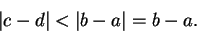This result should be clear from the picture. We can give an analytic proof as follows.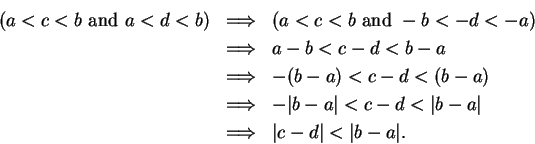6.8   Examples. Let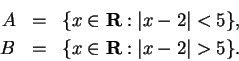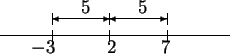Then a numberis in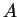if and only if the distance fromto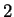is smaller than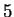, andis in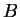if and only if the distance fromtois greater than. I can see by inspection that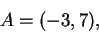and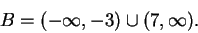Let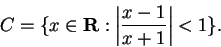If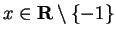, thenis in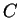if and only if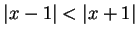, i.e. if and only ifis closer to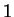than to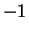.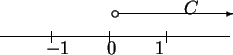I can see by inspection that the point equidistant fromandis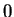, and that the numbers that are closer tothan toare the positive numbers, so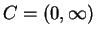. I can also do this analytically, (but in practice I wouldn't) as follows. Since the alsolute values are all non-negative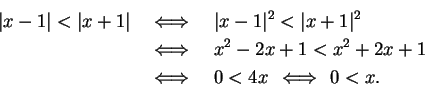6.9   Exercise. Express each of the four sets below as an interval or a union of intervals. (You can do this problem by inspection.)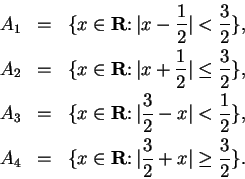6.10   Exercise. Sketch the graphs of the functions from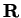todefined by the following equations: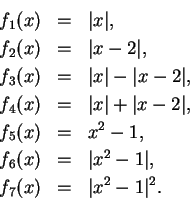(No explanations are expected for this problem.)

6.11   Exercise. Let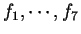be the functions described in the previous exercise. By looking at the graphs, express each of the following six sets in terms of intervals.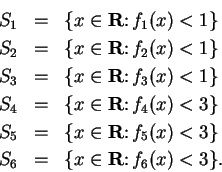Let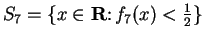. Represent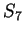graphically on a number line.

Remark: The notationfor absolute value ofwas introduced by Weierstrass in 1841 [Vol 2,page 123]. It was first introduced in connection with complex numbers. It is surprising that analysis advanced so far without introducing a special notation for this very important function.Next: 6.2 Approximation Up: 6. Limits of Sequences Previous: 6. Limits of Sequences   Index
Ray Mayer 2007-09-07